﻿ 用于直下式激光背光模组的衍射光学元件设计 Diffractive Optical Element for Direct-Lit Laser Backlight Unit

Optoelectronics
Vol.08 No.02(2018), Article ID:25631,9 pages
10.12677/OE.2018.82010

Diffractive Optical Element for Direct-Lit Laser Backlight Unit

Zixue Liu, Qibin Feng, Guoqiang Lv*

National Engineering Laboratory of Special Display Technology, National Key Laboratory of Advanced Display Technology, Academy of Photoelectric Technology, Hefei University of Technology, Hefei Anhui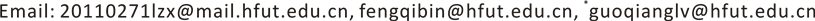Received: Jun. 4th, 2018; accepted: Jun. 20th, 2018; published: Jun. 28th, 2018ABSTRACT

When used in a direct-lit backlight, the ellipse light spot of a laser diode has to be modulated to be rectangular. Aiming at the problem of small divergence angle and long mixing distance, a diffractive optical element for shaping laser light spot is designed by using diffractive optical element algorithm. First, the amplitude distribution of the input and output light fields and the imaging distance are determined. The diffraction formula and Gerchberg-Saxton algorithm are applied to calculate the phase distribution of the DOE in Matlab. Then the obtained phase distribution is input into the simulation software Virtual Lab for simulation. The simulation results show there exists the worse distortion on the spot and the power efficiency is not very high. The output light field distribution is modified and the DOE is then redesigned. The results show that the optimized DOE can effectively shape the elliptical light spot emitted by the LD into a rectangular spot of 14 mm × 11 mm. The energy efficiency is improved to 56.08% from the original 46.69%. The uniformity is improved to 87.16% from 80.59%. The design of the DOE in this paper can realize the shaping requirements of laser beam from circular Gauss beam to rectangular uniform spot, satisfying the requirements of a direct-lit backlight unit on luminance and uniformity.

Keywords:Laser Backlight, Beam Shaping, Diffractive Optical Element, Gerchberg-Saxton Algorithm1. 引言

2. 直下式激光背光模组结构

2.1. 现有直下式LED背光模组结构

2.2. 直下式LD背光模组结构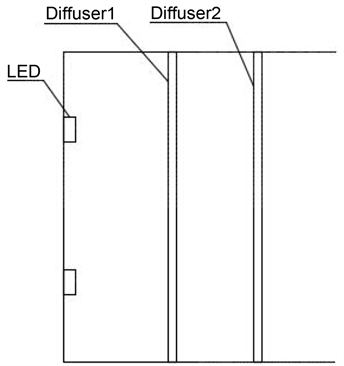Figure 1. Direct-lit LED backlight unit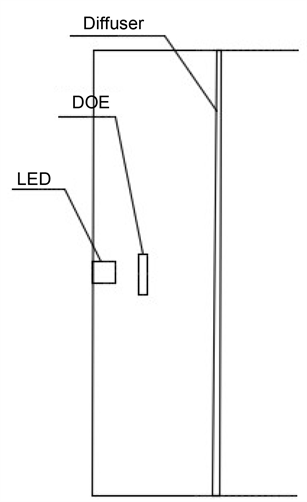Figure 2. Direct-lit laser backlight unit

3. 衍射光学元件设计理论

3.1. 理论基础

$g\left({x}_{1},{y}_{1}\right)={g}_{0}\left({x}_{1},{y}_{1}\right)\ast t\left({x}_{1},{y}_{1}\right)$ (1)

$u\left({x}_{2},{y}_{2}\right)=\frac{1}{i\lambda }\int {\int }_{-\infty }^{\infty }g\left({x}_{1},{y}_{1}\right)\frac{\mathrm{exp}\left(ikr\right)}{r}K\left(\theta \right)\text{d}{x}_{1}\text{d}{y}_{1}$ (2)

$r=\sqrt{{\left({x}_{2}-{x}_{1}\right)}^{2}+{\left({y}_{2}-{y}_{1}\right)}^{2}+{z}^{2}}$ (3)

$K\left(\theta \right)=\frac{r+z}{2r}$ (4)

3.2. DOE理论设计

${U}_{in}\left({x}_{1},{y}_{1}\right)=\mathrm{exp}\left[-\left({x}_{1}^{2}+{y}_{1}^{2}\right)/{\omega }^{2}\right]$ (5)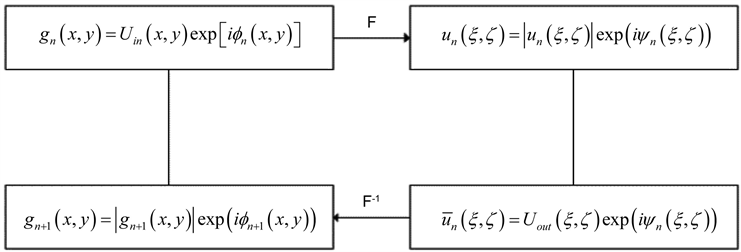Figure 3. GS algorithm flow chart

${U}_{\text{ideal}}\left({x}_{2},{y}_{2}\right)=\left\{\begin{array}{l}1\text{\hspace{0.17em}}\text{\hspace{0.17em}}\text{\hspace{0.17em}}\text{\hspace{0.17em}}\text{ }\text{ }{x}_{2},{y}_{2}\in S\\ 0\text{\hspace{0.17em}}\text{\hspace{0.17em}}\text{\hspace{0.17em}}\text{\hspace{0.17em}}{x}_{2},{y}_{2}\notin S\end{array}$ (6)

4. 软件仿真及优化

${R}_{0}={r}_{0}+d\ast \mathrm{tan}\theta$ (7)

${U}_{in}\left(x,y\right)=\mathrm{exp}\left[-\left(\frac{{x}_{1}^{2}}{{R}_{x}^{2}}+\frac{{y}_{1}^{2}}{{R}_{y}^{2}}\right)\right]$ (8)

$\eta =\frac{{E}_{\text{target}}}{E}$ (9)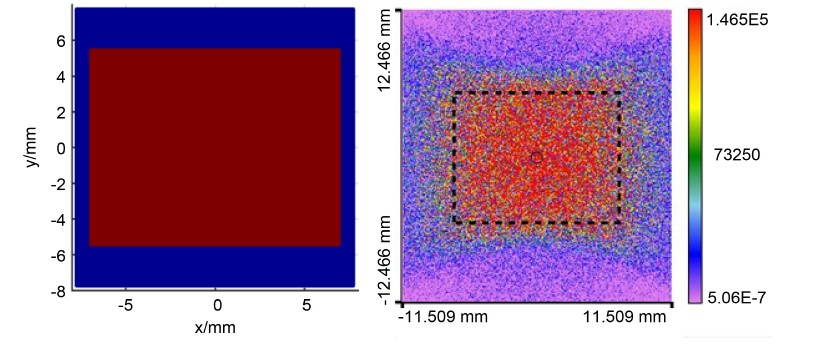Figure 4. Ideal light field distribution and its simulation resultsFigure 5. 9 points

$u={I}_{\mathrm{min}}/{I}_{\text{ave}}$ (10)

$r=z+\frac{{\left({x}_{2}-{x}_{1}\right)}^{2}+{\left({y}_{2}-{y}_{1}\right)}^{2}}{2z}+o\left(\frac{{\left({\left({x}_{2}-{x}_{1}\right)}^{2}+{\left({y}_{2}-{y}_{1}\right)}^{2}\right)}^{2}}{8{z}^{3}}\right)$ (11)

$r=R+\frac{-2{x}_{1}{x}_{2}-2{y}_{1}{y}_{2}+{x}_{1}^{2}+{y}_{1}^{2}}{2R}+o\left(\frac{{\left(-2{x}_{1}{x}_{2}-2{y}_{1}{y}_{2}+{x}_{1}^{2}+{y}_{1}^{2}\right)}^{2}}{8{R}^{3}}\right)$ (12)

$\begin{array}{c}u\left({x}_{2},{y}_{2}\right)=\frac{\mathrm{exp}\left(ikR\right)}{i\lambda z}\frac{z}{R}\int {\int }_{-\infty }^{\infty }g\left({x}_{1},{y}_{1}\right)\mathrm{exp}\left(ik\frac{{x}_{1}^{2}+{y}_{1}^{2}}{R}\right)\\ \text{\hspace{0.17em}}\text{\hspace{0.17em}}×\mathrm{exp}\left[-\frac{ik}{z}\left({x}_{1}\frac{z{x}_{2}}{R}+{y}_{1}\frac{z{x}_{2}}{R}\right)\right]\text{d}{x}_{1}\text{d}{y}_{1}\end{array}$ (13)

$\left\{\begin{array}{l}{x}_{3}=\frac{z}{R}{x}_{2}\\ {y}_{3}=\frac{z}{R}{y}_{2}\\ u\left({x}_{2},{y}_{2}\right)=\frac{z}{R}u\left({x}_{3},{y}_{3}\right)\end{array}$ (14)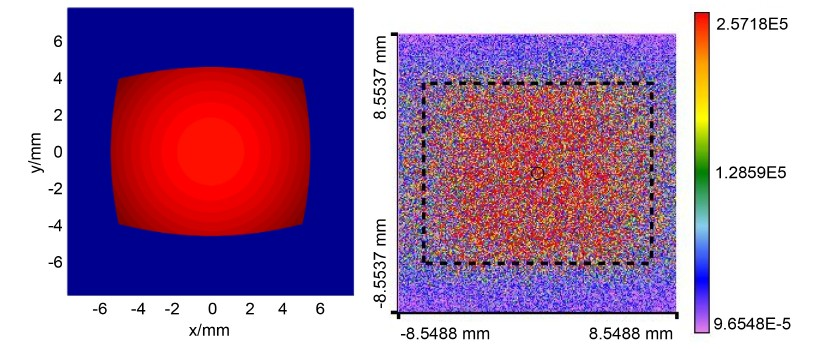Figure 6. The optimized ideal light field distribution and its simulation resultsTable 2. Illumination value of nine points of the optimized design

5. 结论

Diffractive Optical Element for Direct-Lit Laser Backlight Unit[J]. 光电子, 2018, 08(02): 66-74. https://doi.org/10.12677/OE.2018.82010

1. 1. 丁海生. 基于Lcos的激光背投电视的光机设计[D]: [硕士学位论文]. 济南: 山东大学, 2010.

2. 2. 陈旭, 冯玉涛, 刘伟奇, 等. 大屏背投激光显示广角镜头的设计[J]. 光学精密工程, 2011, 19(5): 945-950.

3. 3. 张岳, 郝丽, 柳华, 等. 激光显示的原理与实现[J]. 光学精密工程, 2006, 14(3): 402-405.

4. 4. 梁传样. 激光投影显示光学系统关键技术研究[D]: [博士学位论文]. 长春: 中国科学院长春光学精密机械与物理研究所, 2017.

5. 5. Okuda, Y., Onoda, K., et al. (2012) Laser Backlight Unit Based on a Leaky Optical Fiber. Optical Engineering, 51, 0740011-0740018. https://doi.org/10.1117/1.OE.51.7.074001

6. 6. 孙玉宝. 液晶平板显示激光背光模组的设计与实验研究[D]: [硕士学位论文]. 济南: 山东大学, 2013.

7. 7. 王志平, 曹银花, 刘友强, 等. 蓝光LD平板显示背光模组设计[J]. 中国激光, 2017, 44(5): 0505003.

8. 8. 赵致童, 张贺, 邹永刚, 等. 液晶显示器激光背光光源侧体发光光纤的设计与研究[J]. 中国激光, 2017, 44(3): 0301004.

9. 9. 张巍, 梁传样, 李金, 等. 用于激光数字投影显示系统的匀光整形元件设计[J]. 光学学报, 2015, 35(8): 44-49.

10. 10. 李森森, 吴凡, 吕志伟. 基于液晶空间光调制器的激光近场光束整形[J]. 光电技术应用, 2017, 32(3): 19-23.

11. 11. 梁传, 张巍, 芮大为, 等. 基于硅基液晶实现激光投影显示系统匀光整形和散斑抑制[J]. 中国激光, 2016, 43(12): 20-26.

12. 12. 田志辉, 刘伟奇, 李霞, 等. 激光显示中散斑的减弱[J]. 光学精密工程, 2007, 15(9): 1366-1370.

13. 13. 颜树华. 衍射微光学设计[M]. 北京: 国防工业出版社, 2011.

14. 14. 李剑，王海燕，李强，等. SJ/T 11348-2006数字电视平板显示器测量方法[S].

15. 15. 庞辉, 张满, 邓启凌, 等. 基于瑞利-索末菲积分的大衍射角衍射光学元件设计方法[J]. 光子学报, 2015, 44(5): 173-178.

NOTES

*通讯作者。